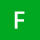Home
IT Knowledge
Inspiration
Languages
EN

# Python - convert camelCase to sentence case

0 points
Created by:Faith-W
413

In this article, we would like to show you how to convert camelCase to sentence case in Python.

## Practical example

In this example, we use `re.sub()` function to replace every capital letter with the same letter in lowercase followed by a space character. Then with string slicing we convert whole string to the lowercase except the first letter which we convert to the uppercase.

``````import re

def sentence_case(string):
if string != '':
result = re.sub('([A-Z])', r' \1', string)
return result[:1].upper() + result[1:].lower()
return

text = "mySampleText"
print(sentence_case(text))``````

Output:

``My sample text``